# How Exactly UMAP Works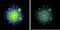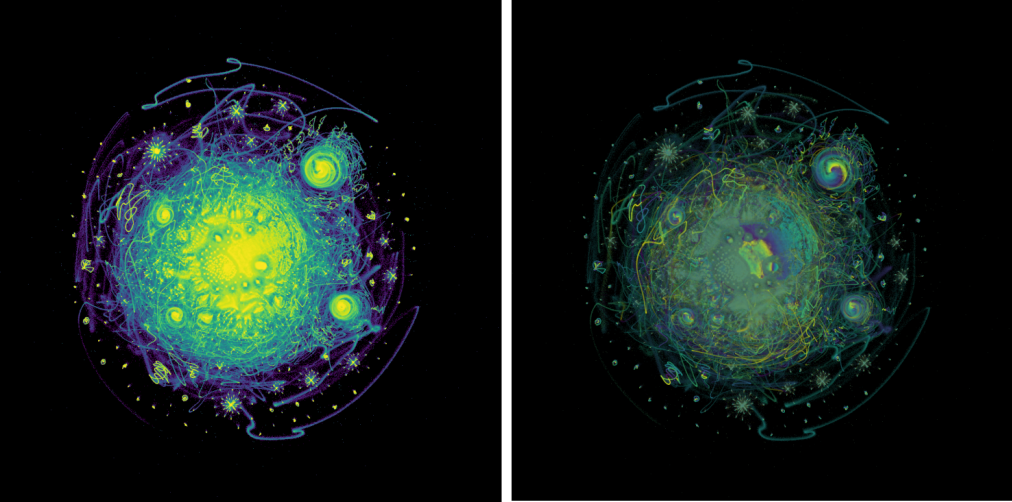This is the twelfth post in the column Mathematical Statistics and Machine Learning for Life Sciences where I try to cover analytical techniques common for Bioinformatics, Biomedicine, Genetics etc. Today we are going to dive into an exciting dimension reduction technique called UMAP that dominates the Single Cell Genomics nowadays. Here, I will try to question the myth about UMAP as a too mathematical method, and explain it using simple language. In the next post, I will show how to program UMAP from scratch in Python, and (bonus!) how to create a dimension reduction technique that provides a better visualization than UMAP. However, now we are going to start slowly with intuition behind UMAP and emphasize key differences between tSNE and UMAP.

# tSNE is Dead. Long Live UMAP!

If you do not know what tSNE is, how it works, and did not read the original revolutionary van der Maaten & Hinton paper from 2008, you probably do not need to know because tSNE is basically dead by now. Despite tSNE made a dramatic impact for the Single Cell Genomics and Data Science in general, it is widely recognized to have a few disadvantages which have to be fixed sooner or later.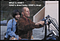What is it exactly that makes us uncomfortable using tSNE for Single Cell genomics? Here I summarize a few points with short comments:

• tSNE does not scale well for rapidly increasing sample sizes in scRNAseq. Attempts to speed it up with FItSNE lead to large memory consumption making it impossible to do the analysis outside of computer cluster, see my banchmarking for 10X Genomics Mouse Brain 1.3M data set.

• tSNE does not preserve global data structure, meaning that only within cluster distances are meaningful while between cluster similarities are not guaranteed, therefore it is widely acknowledged that clustering on tSNE is not a very good idea.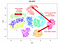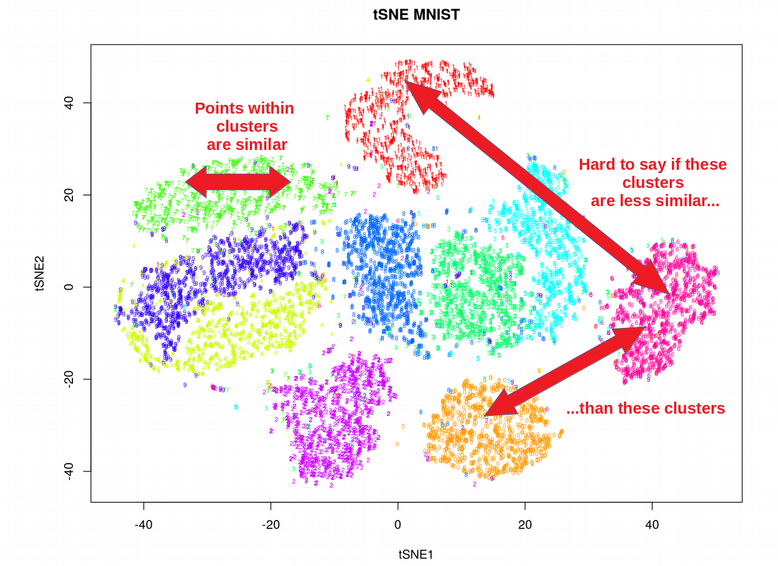• tSNE can practically only embed into 2 or 3 dimensions, i.e. only for visualization purposes, so it is hard to use tSNE as a general dimension reduction technique in order to produce e.g. 10 or 50 components. Please note, this is still a problem for the more modern FItSNE algorithm.

• tSNE performs a non-parametric mapping from high to low dimensions, meaning that it does not leverage features (aka PCA loadings) that drive the observed clustering.

• tSNE can not work with high-dimensional data directly, Autoencoder or PCA are often used for performing a pre-dimensionality reduction before plugging it into the tSNE
• tSNE consumes too much memory for its computations which becomes especially obvious when using large perplexity hyperparameter since the k-nearest neighbor initial step (like in Barnes-Hut procedure) becomes less efficient and important for time reduction. This problem is not solved by the more modern FItSNE algorithm either.

# Brief Recap on How tSNE Works

tSNE is a relatively simple Machine Learning algorithm which can be covered by the following four equations: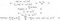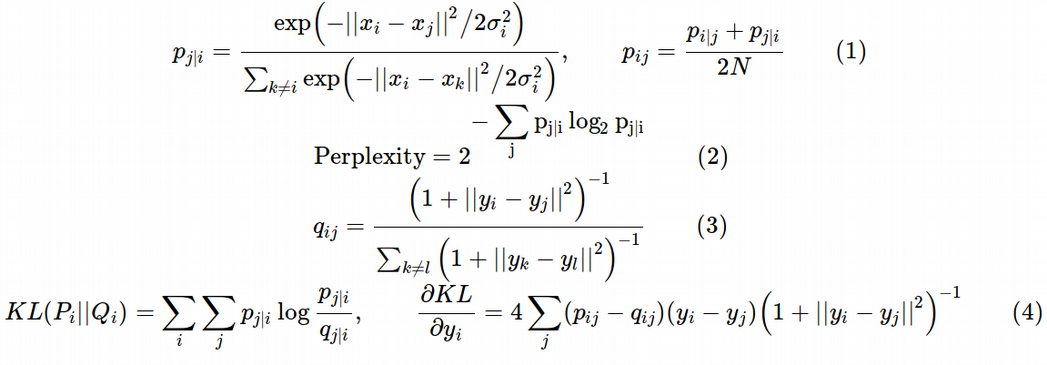Eq. (1) defines the Gaussian probability of observing distances between any two points in the high-dimensional space, which satisfy the symmetry rule. Eq.(2) introduces the concept of Perplexity as a constraint that determines optimal σ for each sample. Eq.(3) declares the Student t-distribution for the distances between the pairs of points in the low-dimensional embedding. The heavy tails of the Student t-distribution are here to overcome the Crowding Problem when embedding into low dimensions. Eq. (4) gives the Kullback-Leibler divergence loss function to project the high-dimensional probability onto the low-dimensional probability, and the analytical form of the gradient to be used in the Gradient Descent optimization.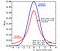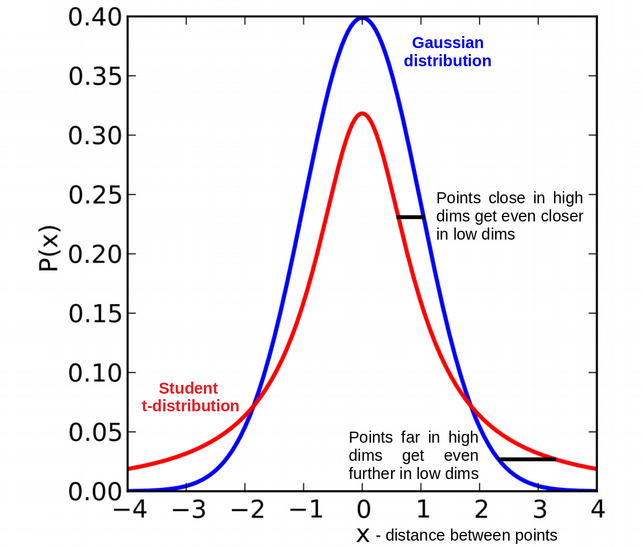Just looking at the figure above I would say that the heavy tails of the Student t-distribution are supposed to provide the global distance information as they push the points far apart in the high dimensions to be even further apart in the low dimensions. However, this good intention is killed by the choice of the cost function (KL-divergence), we will see later why.

# Key Differences Between tSNE and UMAP

My first impression when I heard about UMAP was that this was a completely novel and interesting dimension reduction technique which is based on solid mathematical principles and hence very different from tSNE which is a pure Machine Learning semi-empirical algorithm. My colleagues from Biology told me that the original UMAP paper was “too mathematical”, and looking at the Section 2 of the paper I was very happy to see strict and accurate mathematics finally coming to Life and Data Science. However, reading the UMAP docs and watching Leland McInnes talk at SciPy 2018, I got puzzled and felt like UMAP was another neighbor graph technique which is so similar to tSNE that I was struggling to understand how exactly UMAP is different from tSNE.

From the UMAP paper, the differences between UMAP and tSNE are not very visible even though Leland McInnes tries to summarize them in the Appendix C. I would rather say, I do see small differences but it is not immediately clear why they bring such dramatic effects at the output. Here I will first summarize what I noticed is different between UMAP and tSNE and then try to explain why these differences are important and figure out how large their effects are.

• UMAP uses exponential probability distribution in high dimensions but not necessarily Euclidean distances like tSNE but rather any distance can be plugged in. In addition, the probabilities are not normalized: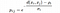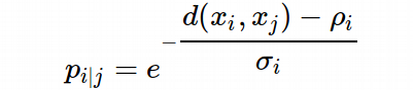Here ρ is an important parameter that represents the distance from each i-th data point to its first nearest neighbor. This ensures the local connectivity of the manifold. In other words, this gives a locally adaptive exponential kernel for each data point, so the distance metric varies from point to point.

The ρ parameter is the only bridge between Sections 2 and 3 in the UMAP paper. Otherwise, I do not see what the fuzzy simplicial set construction, i.e. the fancy topological data analysis from the Section 2, has to do with the algorithmic implementation of UMAP from the Section 3, as it seems at the end of the day the fuzzy simplicial sets lead to just nearest neighbor graph construction.

• UMAP does not apply normalization to either high- or low-dimensional probabilities, which is very different from tSNE and feels weird. However, just from the functional form of the high- or low-dimensional probabilities one can see that they are already scaled for the segment [0, 1] and it turns out that the absence of normalization, like the denominator in Eq. (1), dramatically reduces time of computing the high-dimensional graph since summation or integration is a computationally expensive procedure. Think about Markov Chain Monte Carlo (MCMC) which basically tries to approximately calculate the integral in the denominator of the Bayes rule.

• UMAP uses the number of nearest neighbors instead of perplexity. While tSNE defined perplexity according to Eq. (2), UMAP defines the number of nearest neighbor k without the log2 function, i.e. as follows: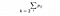• UMAP uses a slightly different symmetrization of the high-dimensional probability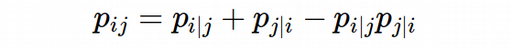The symmterization is necessary since after UMAP glues together points with locally varying metrics (via the parameter ρ), it can happen that the weight of the graph between A and B nodes is not equal to the weight between B and A nodes. Why exactly UMAP uses this kind of symmetrization instead of the one used by tSNE is not clear. My experimentation with different symmetrization rules which I will show in the next post (programming UMAP from scratch) did not convince me that this was such an important step as it had a minor effect on the final low-dimensional embeddings.

• UMAP uses the family of curves 1 / (1+a*y^(2b)) for modelling distance probabilities in low dimensions, not exactly Student t-distribution but very-very similar, please note that again no normalization is applied: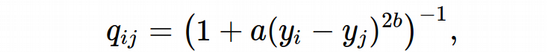where a≈1.93 and b≈0.79 for default UMAP hyperparameters (in fact, for min_dist = 0.001). In practice, UMAP finds a and b from non-linear least-square fitting to the piecewise function with the min_dist hyperparameter: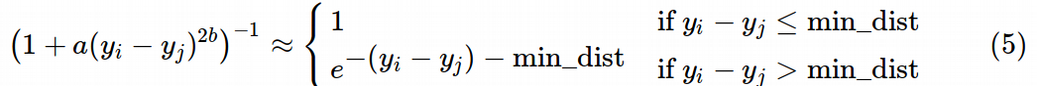To understand better how the family of curves 1 / (1+a*y^(2b)) behaves let us plot a few of the curves for different a and b: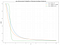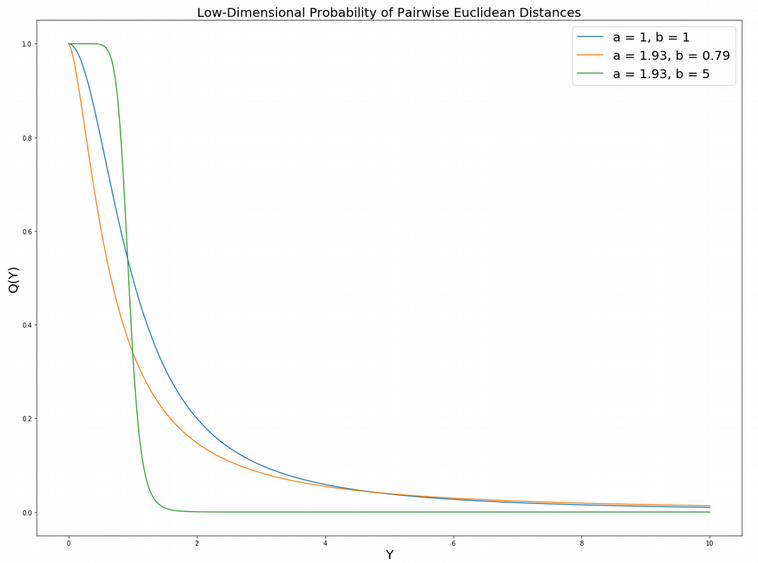We can see that the family of curves is very sensitive to the parameter b, at large b it forms a sort of plateau at small Y. This implies that below the UMAP hyperparameter min_dist all data points are equally tightly connected. Since the Q(Y) function behaves almost like a Heaviside step function it means that UMAP assigns almost the same low-dimensional coordinate for all points that are close to each other in the low-dimensional space. The min_dist is exactly what leads to the super-tightly packed clusters often observed in the UMAP dimensionality reduction plots.

To demonstrate how exactly the a and b parameters are found, let us display a simple piecewise function (where the plateau part is defined via the min_dist parameter) and fit it using the family of functions 1 / (1+a*y^(2b)) by means of optimize.curve_fit from Scipy Python library. As a result of the fit, we obtain the optial a and b parameters for the function 1 / (1+a*y^(2b)).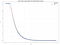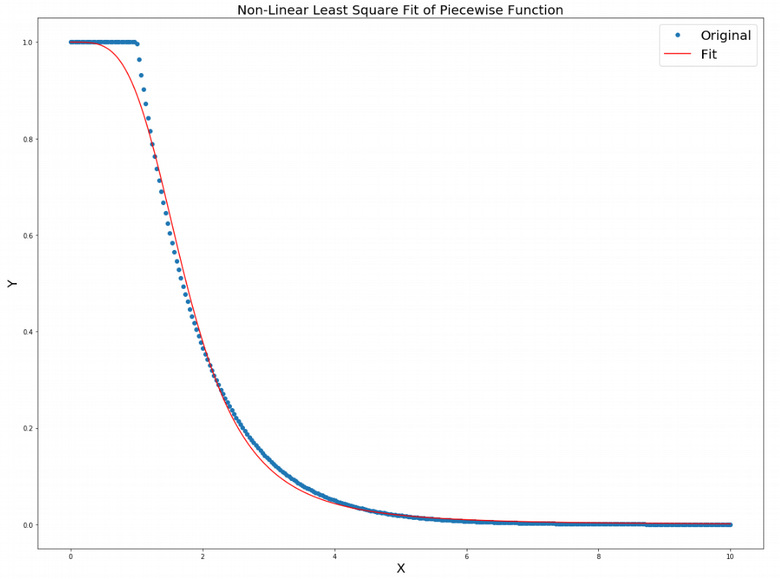• UMAP uses binary cross-entropy (CE) as a cost function instead of the KL-divergence like tSNE does.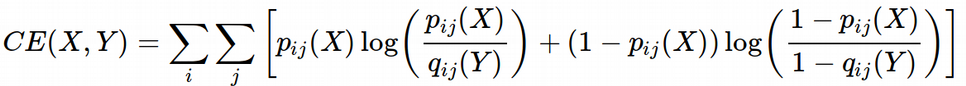In the next section we will show that this additional (second) term in the CE cost function makes UMAP capable of capturing the global data structure in contrast to tSNE that can only model the local structure at moderate perplexity values. Since we need to know the gradient of the cross-entropy in order to implement later the Gradient Descent, let us quickly calculate it. Ignoring the constant terms containing only p(X), we can rewrite the cross-entropy and differentiate it as follows: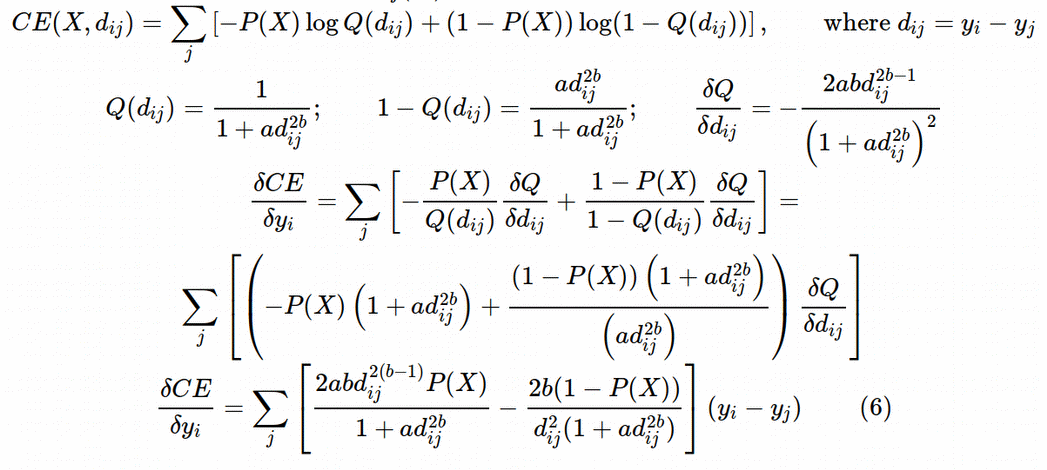• UMAP assigns initial low-dimensional coordinates using Graph Laplacian in contrast to random normal initialization used by tSNE. This, however, should make a minor effect for the final low-dimensional representation, this was at least the case for tSNE. However, this should make UMAP less changing from run to run since it is not a random initialization anymore. The choice of doing initialization through Graph Laplacian is motivated by the interesting hypothesis of Linderman and Steinerberger who suggested that minimization of KL-divergence in the initial stage of tSNE with early exaggeration is equivalent to constructing the Graph Laplacian.

Graph Laplacian, Spectral Clustering, Laplacian Eignemaps, Diffusion Maps, Spectral Embedding, etc. refer to practically the same interesting methodology that combines Matrix Factorization and Neighbor Graph approaches to the dimension reduction problem. In this methodology, we start with constructing a graph (or knn-graph) and formalize it with matrix algebra (adjacency and degree matrices) via constructing the Laplacian matrix, finally we factor the Laplacian matrix, i.e. solving the eigen-value-decomposition problem.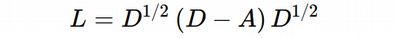We can use the scikit-learn Python library and easily display the initial low-dimensional coordinates using the SpectralEmbedding function on a demo data set which is the Cancer Associated Fibroblasts (CAFs) scRNAseq data: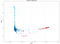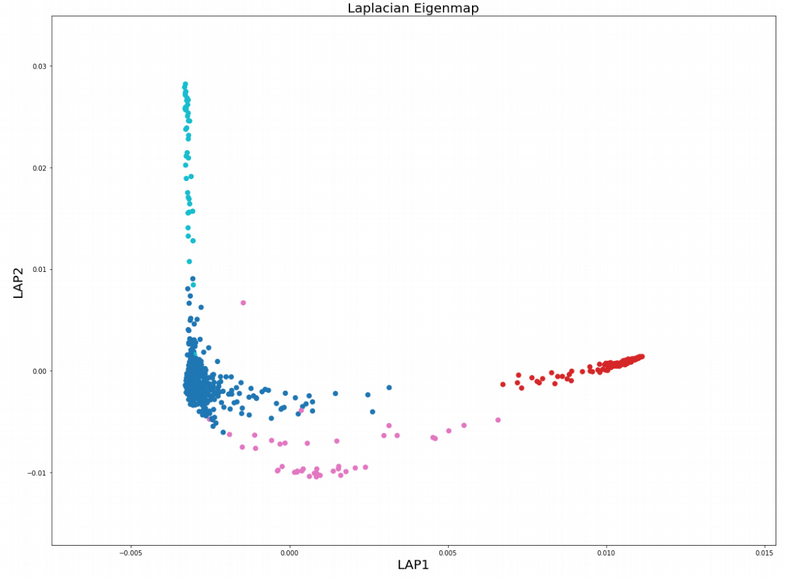• Finally, UMAP uses the Stochastic Gradient Descent (SGD) instead of the regular Gradient Descent (GD) like tSNE / FItSNE, this both speeds up the computations and consumes less memory.

# Why tSNE Preserves Only Local Structure?

Now let us briefly discuss why exactly they say that tSNE preserves only local structure of the data. Locality of tSNE can be understood from different points of view. First, we have the σ parameter in the Eq. (1) that sets how locally the data points “feel” each other. Since the probability of the pairwise Euclidean distances decays exponentially, at small values of σ, it is basically zero for distant points (large X) and grows very fast only for the nearest neighbors (small X). In contrast, at large σ, the probabilities for distant and close points become comparable and in the limit σ→∞, the probability becomes equal to 1 for all distances between any pair of points, i.e. points become equidistant.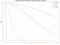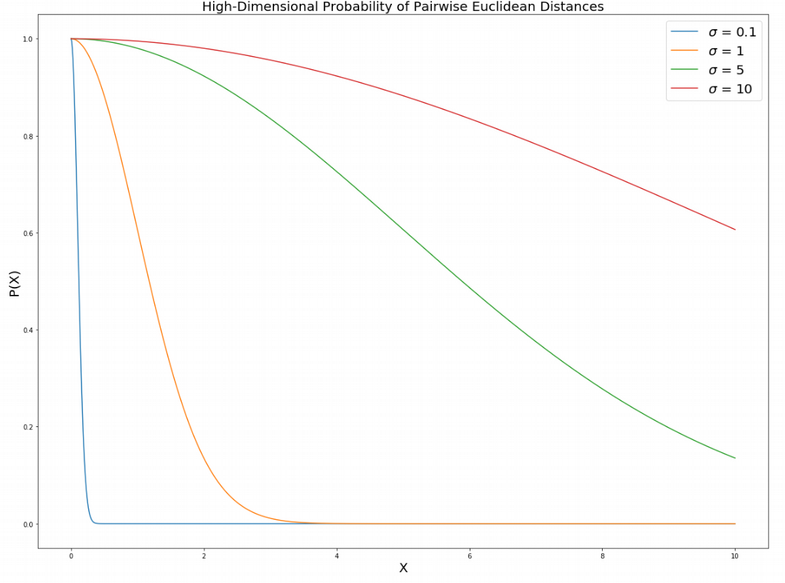Interestingly, if we expand the probability of pairwise Euclidean distances in high dimensions into Taylor series at σ→∞, we will get the power law in the second approximation: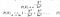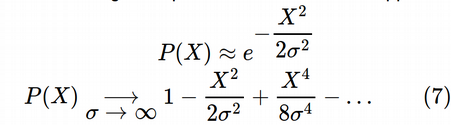The power law with respect to the pairwise Euclidean distances resembles the cost function for the Multi-Dimensional Scaling (MDS) which is known to preserve global distances by trying to preserve distances between each pair of points regardless of whether they are far apart or close to each other. One can interpret this as at large σ tSNE does account for long-range interactions between the data points, so it is not entirely correct to say that tSNE can handle only local distances. However, we typically restrict ourselves by finite values of perplexity, Laurens van der Maaten recommends perplexity values between 5 and 50, although perhaps a good compromise between local and global information would be to select perplexity approximately following the square root law ≈N^(1/2), where N is the sample size. In the opposite limit, σ → 0, we end up with the extreme “locality” in the behaviour of the high-dimensional probability which resembles the Dirac delta-function behavior.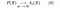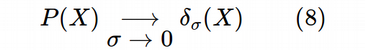Another way to understand the “locality” of tSNE is to think about the KL-divergence function. Let us try to plot it assuming X is a distance between points in high-dimensional space and Y is a low-dimensional distance: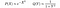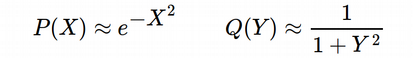From the definition of the KL-divergence, Eq. (4):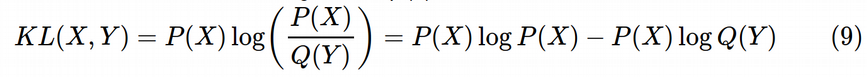The first term in Eq. (9) is close to zero for both large and small X. It goes to zero for small X since the exponent becomes close to 1 and log(1)=0. For large X this term still goes to zero because the exponential pre-factor goes faster to zero than the logarithm goes to −∞. Therefore, for intuitive understanding of the KL-divergence it is enough to to consider only the second term: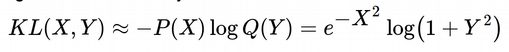This is a weird looking function, let us plot KL(X, Y):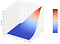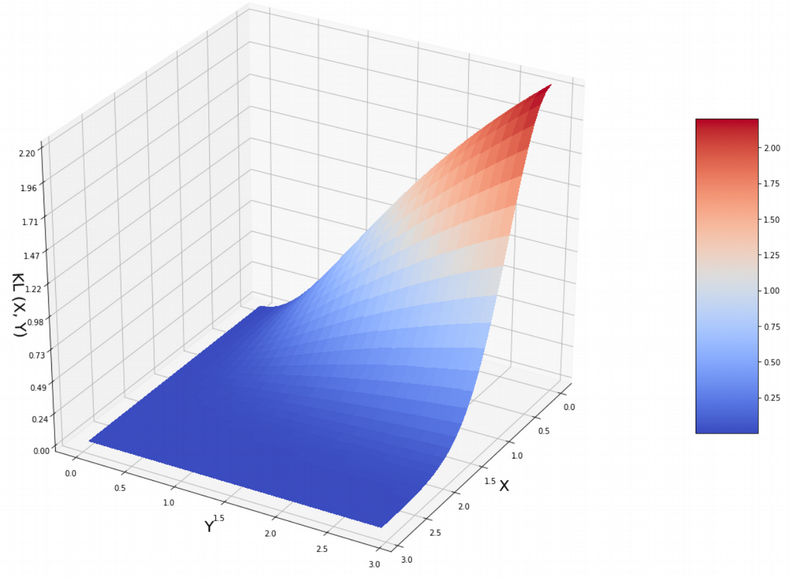The function has a very asymmetric shape. If the distance between the points in high dimensions X is small, the exponential pre-factor becomes 1 and the logarithmic term behaves as log(1+Y^2) meaning that if the distance in low dimensions Y is large, there will be a large penalty, therefore tSNE tries to reduce Y at small X in order to reduce the penalty. In contrast, for large distances X in high dimensions, Y can be basically any value from 0 to ∞ since the exponential term goes to zero and always wins over the logarithmic term. Therefore it might happen that points far apart in high dimensions end up close to each other in low dimensions. Hence, in other words, tSNE does not guarantee that points far apart in high dimensions will be preserved to be far apart in low dimensions. However, it does guarantee that points close to each other in high dimensions will remain close to each other in low dimensions. So tSNE is not really good at projecting large distances into low dimensions, so it preserves only the local data structure provided that σ does not go to ∞.

# Why UMAP Can Preserve Global Structure

In contrast to tSNE, UMAP uses Cross-Entropy (CE) as a cost function instead of the KL-divergence: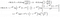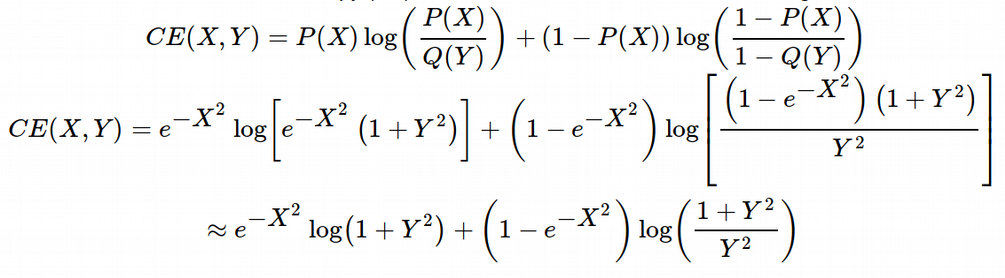This leads to huge changes in the local-global structure preservation balance. At small values of X we get the same limit as for tSNE since the second term disappears because of the pre-factor and the fact that log-function is slower than polynomial function: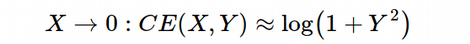Therefore the Y coordinates are forced to be very small, i.e. Y → 0, in order to minimize the penalty. This is exactly like the tSNE behaves. However, in the opposite limit of large X, i.e. X→∞, the first term disappears, pre-factor of the second term becomes 1 and we obtain: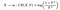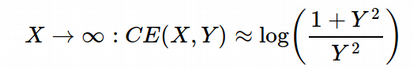Here if Y is small, we get a high penalty because of the Y in the denominator of the logarithm, therefore Y is encouraged to be large so that the ratio under logarithm becomes 1 and we get zero penalty. Therefore we get Y → ∞ at X → ∞, so the global distances are preserved when moving from high- to low-dimensional space, exactly what we want. To demonstrate this, let us plot the UMAP CE cost function: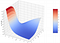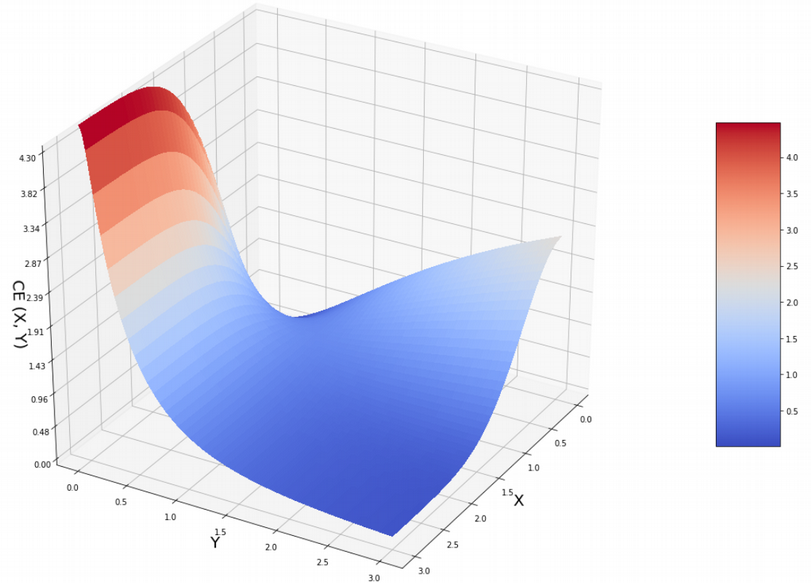Here, we can see that the “right” part of the plot looks fairly similar to the KL-divergence surface above. This means that at low X we still want to have low Y in order to reduce the penalty. However, at large X, the Y distance really wants to be large too, because if it is small, the CE (X, Y) penalty will be enormous. Remember, previously, for KL (X, Y) surface, we did not have any difference in penalty between low and high Y at large X. That is why CE (X, Y) cost function is capable of preserving global distances as well as local distances.

# Why Exactly UMAP is Faster than tSNE

We know that UMAP is faster than tSNE when it concerns a) large number of data points, b) number of embedding dimensions greater than 2 or 3, c) large number of ambient dimensions in the data set. Here, let us try to understand how superiority of UMAP over tSNE comes from the math and the algorithmic implementation.

Both tSNE and UMAP essentially consist of two steps.

• Building a graph in high dimensions and computing the bandwidth of the exponential probability, σ, using the binary search and the fixed number of nearest neighbors to consider.

• Optimization of the low-dimensional representation via Gradient Descent. The second step is the bottleneck of the algorithm, it is consecutive and can not be multi-threaded. Since both tSNE and UMAP do the second step, it is not immediately obvious why UMAP can do it more efficiently than tSNE.

However, I noticed that the fist step became much faster for UMAP than it was for tSNE. This is because of two reasons.

• First, we dropped the log-part in the definition of the number of nearest neighbors, i.e. not using the full entropy like tSNE:Since algorithmically the log-function is computed through the Taylor series expansion, and practically putting a log-prefactor in front of the linear term does not add much since log-function is slower than the linear function, it is nice to skip this step entirely.

• Second reason is that we omitted normalization of the high-dimensional probability, aka one used in Eq. (1) for tSNE. This arguably small step had actually a dramatic effect on the performance. This is because summation or integration is a computational expensive step.

Next, UMAP actually becomes faster on the second step as well. This improvement has also a few reasons:

• Stochastic Gradient Descent (SGD) was applied instead of the regular Gradient Descent (GD) like for tSNE or FItSNE. This improves the speed since for SGD you calculate the gradient from a random subset of samples instead of using all of them like for regular GD. In addition to speed this also reduces the memory consumption since you are no longer obliged to keep gradients for all your samples in the memory but for a subset only.

• We skipped normalization not only for high-dimensional but also for low-dimensional probabilities. This omitted the expensive summation on the second stage (optimizing low-dimensional embeddings) as well.

• Since the standard tSNE uses tree-based algorithms for nearest neighbor search, it is too slow for producing more than 2–3 embedding dimensions since the tree-based algorithms scale exponentially with the number of dimensions. This problem is fixed in UMAP by dropping the normalization in both high- and low-dimensional probabilities.

• Increasing the number of dimensions in the original data set we introduce sparsity on the data, i.e. we get more and more fragmented manifold, i.e. sometimes there are dense regions, sometimes there are isolated points (locally broken manifold). UMAP solves this problem by introducing the local connectivity ρ parameter which glues together (to some extent) the sparse regions via introducing adaptive exponential kernel that takes into account the local data connectivity. This is exactly the reason why UMAP can (theoretically) work with any number of dimensions and does not need the pre-dimensionality reduction step (Autoencoder, PCA) before plugging it into the main dimensionality reduction procedure.

# Summary

In this post, we have learnt that despite tSNE served the Single Cell research area for years, it has too many disadvantages such as speed and the lack of global distance preservation. UMAP overall follows the philosophy of tSNE, but introduces a number of improvements such as another cost function and the absence of normalization of high- and low-dimensional probabilities.

http://wap.sciencenet.cn/blog-2866696-1246304.html

## 全部精选博文导读

GMT+8, 2020-12-2 12:43